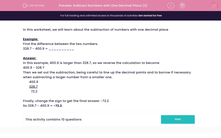# Subtract Numbers with One Decimal Place (2)

In this worksheet, students subtract numbers with one decimal place.This content is premium and exclusive to EdPlace subscribers.Key stage:  KS 2

Curriculum topic:   Maths and Numerical Reasoning

Curriculum subtopic:   Decimals

Difficulty level:#### Worksheet Overview

In this worksheet, we will learn about the subtraction of numbers with one decimal place

Example:

Find the difference between the two numbers.

328.7 - 400.9 = __________

In this example, 400.9 is larger than 328.7, so we reverse the calculation to become

400.9 - 328.7

Then we set out the subtraction, being careful to line up the decimal points and to borrow if necessary when subtracting a larger number from a smaller one.

400.9

328.7

72.2

Finally, change the sign to get the final answer -72.2

So 328.7 - 400.9 = -72.2

### What is EdPlace?

We're your National Curriculum aligned online education content provider helping each child succeed in English, maths and science from year 1 to GCSE. With an EdPlace account you’ll be able to track and measure progress, helping each child achieve their best. We build confidence and attainment by personalising each child’s learning at a level that suits them.

Get started